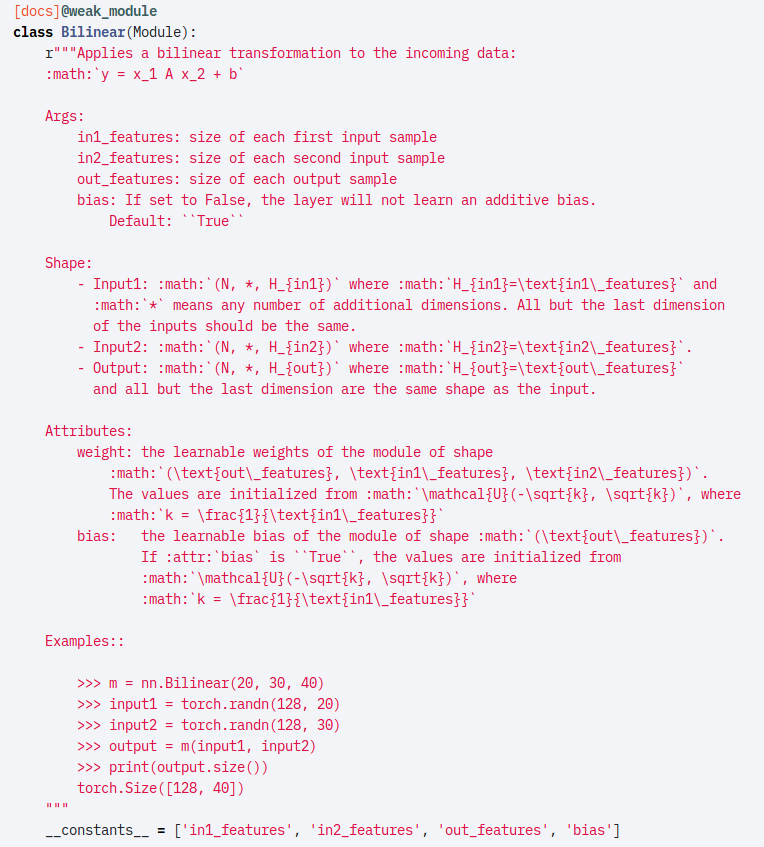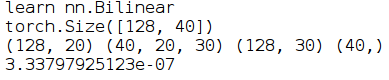## pytorch中的nn.Bilinear的计算原理详解``````print('learn nn.Bilinear')
m = nn.Bilinear(20, 30, 40)
input1 = torch.randn(128, 20)
input2 = torch.randn(128, 30)
output = m(input1, input2)
print(output.size())
arr_output = output.data.cpu().numpy()

weight = m.weight.data.cpu().numpy()
bias = m.bias.data.cpu().numpy()
x1 = input1.data.cpu().numpy()
x2 = input2.data.cpu().numpy()
print(x1.shape,weight.shape,x2.shape,bias.shape)
y = np.zeros((x1.shape,weight.shape))
for k in range(weight.shape):
buff = np.dot(x1, weight[k])
buff = buff * x2
buff = np.sum(buff,axis=1)
y[:,k] = buff
y += bias
dif = y - arr_output
print(np.mean(np.abs(dif.flatten())))``````